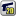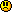﻿ Unreal Software - Thread: Distance between players

# ForumCS2DScriptsDistance between players

#Distance between players

13 replies
Goto Page1Echo-Charlie
User
OfflineHello there. I have an idea, but I can't realise that - I don't know how can I get distance between two players (distance in pixels).Maybe someone of you can explain how can I get distance? Thanks a lot for your answers.DannyDeth
User
OfflineThe Pythagoream Theorem states that the hypotenuse is equal to the adjacent + the opposite squared, so therefore if you find the vector of two points ( x1 - x2, y1 -y2 ) you can find the length of the hypotenuse.

So basically you can do it like this:
Code:
1
distance = math.sqrt(player(id1,"x") - player(id2,"x") + player(id1,"y") - player(id2,"y"))

Just replace id1 and id2 with the variables you are using.
Echo-Charlie
User
Offlinethanks a lot, I'll try your stated code aboveDannyDeth
User
OfflineIt's a pleasure to helpVectarrio
User
Offline@DannyDeth
You didn't "square" the variables:
Code:
1
distance = math.sqrt((player(id1,"x") - player(id2,"x"))^2 + (player(id1,"y") - player(id2,"y"))^2)
Unknown_Soldier
User
OfflineThat is wrong, to calculate the hypotenuse, you need the length of the other 2 sides of the triangle, the code is something like this

Code:
1
2
3
4
side1 = math.max(player(id1,"x"),player(id2,"x"))-math.min(player(id1,"x"),player(id2,"x"))
side2 = math.max(player(id1,"y"),player(id2,"y"))-math.min(player(id1,"y"),player(id2,"y"))

distance = math.sqrt(side1^2+side2^2)

You cant use math.sqrt on a negative number
Should I come back and make one last map for CS2D?
Vectarrio
User
Offline@Unknown_Soldier
I don't know, but my version worked normal for me.Unknown_Soldier
User
OfflineLet's try your code with an example

player 1 X100 Y100

player 2 X200 Y300

sqrt(100 - 200 ^ 2 + 100 - 300 ^ 2)

sqrt(100 - 40000 + 100 - 90000)

sqrt(-39900 - 89900)

sqrt(-129800)

no number multiplyed by himself can be a negative number

example

2^2 = 2*2 = 4

-2^2 = -2*-2 = 4
Should I come back and make one last map for CS2D?
Vectarrio
User
OfflineDon't you see that I put brackets?
Unknown_Soldier
User
Offline@Vectar666 No, I didn't see them. Sry, I have problems with brackets when I make luas. Anyway, both codes should work.
Should I come back and make one last map for CS2D?
Echo-Charlie
User
Offlinethanks a lot. It worked for meVectarrio
User
OfflineUnknown_Soldier has written:
@Vectar666 No, I didn't see them. Sry, I have problems with brackets when I make luas. Anyway, both codes should work.

and, I'd prefer math.abs instead of math.max with math.min.
Just a little less space eaten by it.archmage
User
OfflineJust use this
Code:
1
2
3
4
5
6
function math.dist(p1, p2)
local x, y = player(p1, "x"), player(p2, "y");
local x2, y2 = player(p2, "x"), player(p2, "y");

return math.sqrt((y2-y)^2 + (x2-x)^2);
end

Just call it with two player IDs.
Ex.:
Code:
1
dist = math.dist(1, 32);
We must secure the existence of our people and a future for white children. 14/88
DannyDeth
User
OfflineVectar666 has written:
You didn't "square" the variables

Fudge cakes. It was late and I was tired, what can I say?1﻿